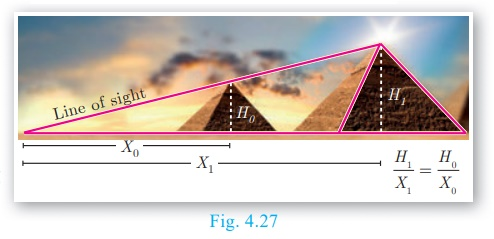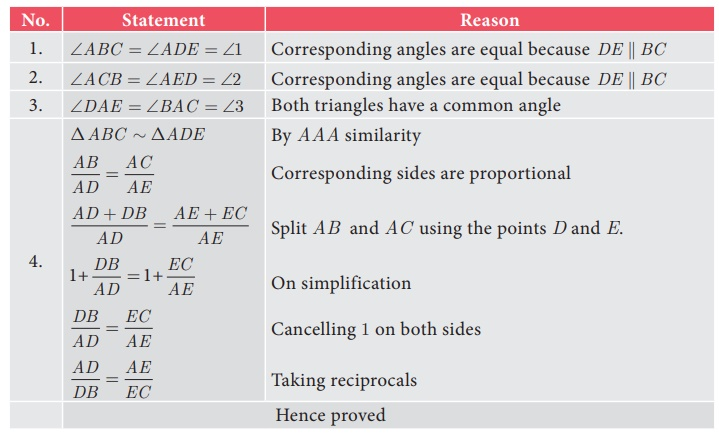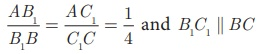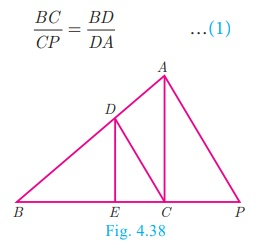Home | | Maths 10th Std | Thales Theorem and Angle Bisector Theorem

# Thales Theorem and Angle Bisector Theorem

Theorem 1: Basic Proportionality Theorem (BPT) or Thales theorem, Theorem 2: Converse of Basic Proportionality Theorem, Theorem 3: Angle Bisector Theorem, Theorem 4: Converse of Angle Bisector Theorem

Thales Theorem and Angle Bisector Theorem

## Introduction

Thales, (640 - 540 BC (BCE)) the most famous Greek mathematician and philosopher lived around seventh century BC (BCE). He possessed knowledge to the extent that he became the first of seven sages of Greece. Thales was the first man to announce that any idea that emerged should be tested scientifically and only then it can be accepted. In this aspect, he did great investigations in mathematics and astronomy and discovered many concepts. He was credited for providing first proof in mathematics, which today is called by the name “Basic Proportionality Theorem”. It is also called “Thales Theorem” named after its discoverer.The discovery of the Thales theorem itself is a very interesting story. When Thales travelled to Egypt, he was challenged by Egyptians to determine the height of one of several magnificent pyramids that they had constructed. Thales accepted the challenge and used similarity of triangles to determine the same successfully, another triumphant application of Geometry. Since X0, X1 and H0 are known, we can determine the height H1 of the pyramid.

To understand the basic proportionality theorem or Thales theorem, let us do the following  activity.## Basic Proportionality Theorem (BPT) or Thales theorem

### Statement

A straight line drawn parallel to a side of triangle intersecting the other two sides, divides the sides in the same ratio.### Proof

Given: In ΔABC , D is a point on AB and E is a point on AC.

### To prove:### Construction:

Draw a line DE || BCCorollary

If in ΔABC , a straight line DE parallel to BC, intersects AB at D and AC at E, then### Proof

In ΔABC, DE || BC.

therefore,=> AD/DB = AE/EC (by Basic Proportionality Theorem)Is the converse of Basic Proportionality Theorem also true? To examine let us do the following illustration.

### Illustration

Draw an angle XAY on your notebook as shown in Fig.4.31 and on ray AX, mark points B1 , B2 , B 3 , B4 and B such that AB1 = B1B2 = B2 B3 = B 3 B4 = B 4 B = 1 cm.

Similarly on ray AY, mark points C1, C2 , C3 ,C4  and C, such that AC1=C1C2 = C2C3 = C3C4 = C4C = 2 cm, Join B1 C1  and BC

Observe thatSimilarly joining B2C2, B3C3 and B4C4 you see thatFrom this we observe that if a line divides two sides of a triangle in the same ratio, then the line is parallel to the third side.

Therefore, we obtain the following theorem called converse of the Thales theorem.

Theorem 2:

## Converse of Basic Proportionality Theorem

### Statement

If a straight line divides any two sides of a triangle in the same ratio, then the line must be parallel to the third side.

### Proof

Given : In  ABCTo prove : DE  || BC

Construction Draw BF || DE## Angle Bisector Theorem

### Statement

The internal bisector of an angle of a triangle divides the opposite side internally in the ratio of the corresponding sides containing the angle.

### Proof

Given :  In ΔABC, AD is the internal bisector

To prove :Construction : Draw a line through C parallel to AB. Extend AD to meet line through C at ETheorem 4:

## Converse of Angle Bisector Theorem

### Statement

If a straight line through one vertex of a triangle divides the opposite side internally in the ratio of the other two sides, then the line bisects the angle internally at the vertex.

### Proof

Given : ABC is a triangle. AD divides BC in the ratio of the sides containing the angles A to meet BC at D.

That isTo prove : AD bisects A      i.e. 1 = 2

Construction : Draw CE || DA . Extend BA to meet at E.Example 4.12

In ΔABC , if DE || BC , AD = x,  DB = x 2,  and EC = x −1 then find the lengths of the sides AB and AC.

Solution

In ΔABC we have DE || BC .

By Thales theorem, we havex/ x-2 = (x + 2)/(x - 1) gives x (x − 1) = (x − 2)(x + 2)

Hence, x 2x = x2 – 4 so, x = 4

When x = 4 , AD = 4 , DB = x − 2 = 2 , AE = x + 2 = 6 , EC = x – 1 =3

Hence, AB = AD + DB = 4 + 2 = 6 , AC = AE + EC = 6 + 3 = 9 .

Therefore, AB = 6,  AC = 9.

### Example 4.13

D and E are respectively the points on the sides AB and AC of a ΔABC such that AB=5.6 cm, AD=1.4 cm, AC=7.2 cm and AE = 1.8 cm, show that DE || BC.

### Solution

We have AB = 5.6    cm, AD =1.4    cm, AC  7.2 cm and AE = 1.8 cm.

BD = AB AD = 5.6–1.4 = 4.2 cm

and EC = ACAE  = 7.2–1.8 = 5.4 cm.Therefore, by converse of Basic Proportionality Theorem, we have DE is parallel to BC.

Hence proved.

### Example 4.14

In the Fig.4.38, DE || AC and DC || AP . Prove that.

### Solution

Solution In ΔBPA, we have DC || AP . By Basic Proportionality Theorem,

we haveIn ΔBCA, we have DE || AC . By Basic Proportionality Theorem, we haveFrom (1) and (2) we get, BE/EC = BC/CP =>. Hence proved.

### Example 4.15

In the Fig.4.39, AD is the bisector of A . If BD = 4 cm, DC = 3 cm and AB = 6 cm, find AC.

### Solution

In ΔABC , AD is the bisector of

Therefore by Angle Bisector Theorem4/3 = 6/ AC

gives 4AC = 8 . Hence, AC = 9/2 =4.5 cm

### Example 4.16

In the Fig. 4.40, AD is the bisector of BAC , if AB = 10 cm, AC = 14 cm and BC = 6 cm. Find BD and DC

### Solution

Let BD = x cm, then DC = (6–x)cm

AD is the bisector of A

Therefore by Angle Bisector TheoremTherefore, BD = 2.5 cm,   DC = 6 x = 6 2.5 = 3.5 cm

Tags : Statement, Proof, Construction, Illustration, Solved Example Problems , 10th Mathematics : UNIT 4 : Geometry
Study Material, Lecturing Notes, Assignment, Reference, Wiki description explanation, brief detail
10th Mathematics : UNIT 4 : Geometry : Thales Theorem and Angle Bisector Theorem | Statement, Proof, Construction, Illustration, Solved Example Problems